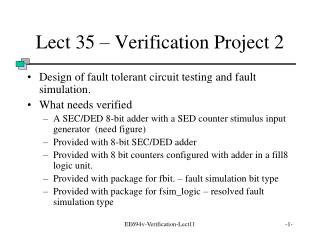Download PresentationLect 35 – Verification Project 2

# Lect 35 – Verification Project 2

Download Presentation## Lect 35 – Verification Project 2

- - - - - - - - - - - - - - - - - - - - - - - - - - - E N D - - - - - - - - - - - - - - - - - - - - - - - - - - -
##### Presentation Transcript

1. Lect 35 – Verification Project 2 • Design of fault tolerant circuit testing and fault simulation. • What needs verified • A SEC/DED 8-bit adder with a SED counter stimulus input generator (need figure) • Provided with 8-bit SEC/DED adder • Provided with 8 bit counters configured with adder in a fill8 logic unit. • Provided with package for fbit. – fault simulation bit type • Provided with package for fsim_logic – resolved fault simulation type EE694v-Verification-Lect11

2. The SEC/DED adder • In general the architecture is EE694v-Verification-Lect11

3. Type fbit • Fbit – fault simulation bit type • During evaluation of basic functions like AND, OR, XOR, etc., the output is probablistically the inverse of what it should be, i.e., in error. • Two packages to verify fbit and fsim_logic EE694v-Verification-Lect11

4. Overhead of fault simulation • What is overhead???? • On every gate evaluation a random number is generated. SO LOTS OF OVERHEAD computation. • Part of work is to evaluate that overhead. • How to do it? Run the adder (type bit exhaustive) and have the time. Run the adder (type fbit) and have the time. Run the adder (type fsim_logic) and have the time. Include this in the report. EE694v-Verification-Lect11

5. What may need to be added • Fbit_logic package needs to include a type conversion function to convert to/from type bit. This is such that the testbench can generate the test transactions without error. • DONE but not verified yet. EE694v-Verification-Lect11

6. What to verify • The fault tolerant SEC/DED 8-bit adder • The fbit logic package – the full package • The fsim_logic_package – the full package EE694v-Verification-Lect11

7. The verification plan • Details what is to be verified and how along with the rationale. • In this project are verifying the design, architecture, and HDL coding of the SEC/DED adder. • Additionally you are verifying the two packages for fault injection, fbit and fsim_logic which parallel the existing types bit and std_logic EE694v-Verification-Lect11

8. Fault simulation logic packages • fbit_logic is the package that declares and defines type fbit and fbit_vector. • Corresponds to type bit • Has an error injection threshold • Constant threshold is declared in the package declarative part. • When set to 1 then no errors are injected • When set to .99 then 1 in 100 gate evaluations result in an erroneous output. • Can vary the threshold to obtain meaningful fault simulation in finite time. EE694v-Verification-Lect11

9. Fault simulation stdlogic package • fsim_logic is the package that declares and defines type fsim_logic and fsim_logic_vector • Corresponds to type std_logic • As with type fbit there is an error injection threshold • Models can be modified to use fault simulation types. Only modification needed is to modify the type in the ports and declarations of the designs. EE694v-Verification-Lect11